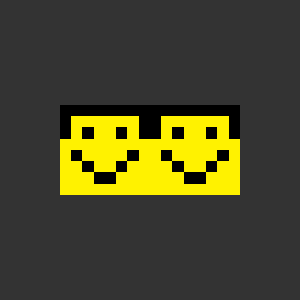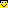# How to repeat texture on SimplePlane?

## Recommended Posts

Hello!

I have a texture 8x8 and a plane 16x8...

How I can repeat the texture?

I tryied this but dont works

texture.baseTexture.wrapMode = PIXI.WRAP_MODES.REPEAT;
##### Share on other sites

it can work only if texture is pow2 or you are using webgl2.

also make sure that UV's spawned by plane are from (0-2) by U, that's 2 repeats

##### Share on other sites

4 hours ago, FlokiTV said:

I have a texture 8x8 and a plane 16x8...

8x8 is a texture whose side is 2 cubes. Works in WebGL 1.0 where texture side must be a power of two, for example: 2x2, 4x4, 8x8, 16x64, 64x32 and so on. I don't understand why this doesn't work in Pixi.js, so I use pure WebGL where I have full control over what happens.The 8x8 texture:index.html

```<html>

<script src="https://cdn.jsdelivr.net/npm/[email protected]/gl-matrix-min.js"></script>

<body>
<canvas id="renderCanvas" width="300" height="300"></canvas>

<script>
// Get the WebGL context
const gl = document.getElementById("renderCanvas").getContext("webgl");

`
attribute vec2 aPosition;
attribute vec2 aTexCoord;
uniform mat4 uMvpMatrix;
varying vec2 vTexCoord;

void main()
{
gl_Position = uMvpMatrix * vec4(aPosition, 0.0, 1.0);
vTexCoord = aTexCoord;
}`;

`
precision mediump float;
varying vec2 vTexCoord;
uniform sampler2D uSampler;
void main()
{
gl_FragColor = texture2D(uSampler, vTexCoord);
}`;

const program = gl.createProgram();
gl.useProgram(program);

// Create a vertex buffer
const vertexPositions = [
-0.5, -0.5,
-0.5, 0.5,
0.5, -0.5,
0.5, 0.5
];
const vertexPosBuffer = gl.createBuffer();
gl.bindBuffer(gl.ARRAY_BUFFER, vertexPosBuffer);
gl.bufferData(gl.ARRAY_BUFFER, new Float32Array(vertexPositions), gl.STATIC_DRAW);
// Setting the position attribute
const aPositionLocation = gl.getAttribLocation(program, "aPosition");
gl.vertexAttribPointer(aPositionLocation, 2, gl.FLOAT, false, 0, 0);
gl.enableVertexAttribArray(aPositionLocation);

// Create a texture coord buffer
const texCoords = [
0, 0,
0, 1,
2, 0,
2, 1
];
const texCoordBuffer = gl.createBuffer();
gl.bindBuffer(gl.ARRAY_BUFFER, texCoordBuffer);
gl.bufferData(gl.ARRAY_BUFFER, new Float32Array(texCoords), gl.STATIC_DRAW);
// Setting the texture coordinates attribute
const aTexCoordLocation = gl.getAttribLocation(program, "aTexCoord");
gl.vertexAttribPointer(aTexCoordLocation, 2, gl.FLOAT, false, 0, 0);
gl.enableVertexAttribArray(aTexCoordLocation);

// Set up the camera and projection
const viewMatrix = glMatrix.mat4.create();
glMatrix.mat4.lookAt(viewMatrix, [0, 0, 90], [0, 0, 0], [0, 1, 0]);
const projMatrix = glMatrix.mat4.create();
glMatrix.mat4.ortho(projMatrix, 0, 100, 100, 0, -100, 100);
const projViewMatrix = glMatrix.mat4.create();
glMatrix.mat4.mul(projViewMatrix, projMatrix, viewMatrix);

// Set object size and object position
const modelMatrix = glMatrix.mat4.create();
glMatrix.mat4.translate(modelMatrix, modelMatrix, [50, 50, 1]);
glMatrix.mat4.scale(modelMatrix, modelMatrix, [60, 30, 1]);

// Send mvp matrix to shader
const uMvpMatrixLocation = gl.getUniformLocation(program, "uMvpMatrix");
const mvpMatrix = glMatrix.mat4.create();
glMatrix.mat4.mul(mvpMatrix, projViewMatrix, modelMatrix);
gl.uniformMatrix4fv(uMvpMatrixLocation, false, mvpMatrix);

const image = new Image();
{
const texture = gl.createTexture();
gl.bindTexture(gl.TEXTURE_2D, texture);
gl.texParameteri(gl.TEXTURE_2D, gl.TEXTURE_MIN_FILTER, gl.NEAREST);
gl.texParameteri(gl.TEXTURE_2D, gl.TEXTURE_MAG_FILTER, gl.NEAREST);
gl.texParameteri(gl.TEXTURE_2D, gl.TEXTURE_WRAP_S, gl.REPEAT);
gl.texParameteri(gl.TEXTURE_2D, gl.TEXTURE_WRAP_T, gl.REPEAT);
gl.texImage2D(gl.TEXTURE_2D, 0, gl.RGB, gl.RGB, gl.UNSIGNED_BYTE, image);
draw();
};
image.crossOrigin = "";
image.src = "./images/smile.png";

// Set a color for background
gl.clearColor(0.2, 0.2, 0.2, 1.0);

function draw()
{
// Clear the background with the selected color
gl.clear(gl.COLOR_BUFFER_BIT);

// Draw an object
gl.drawArrays(gl.TRIANGLE_STRIP, 0, 4);
}
</script>

</body>

</html>```

Edited by 8Observer8

## Join the conversation

You can post now and register later. If you have an account, sign in now to post with your account.
Note: Your post will require moderator approval before it will be visible.×   Pasted as rich text.   Paste as plain text instead

Only 75 emoji are allowed.